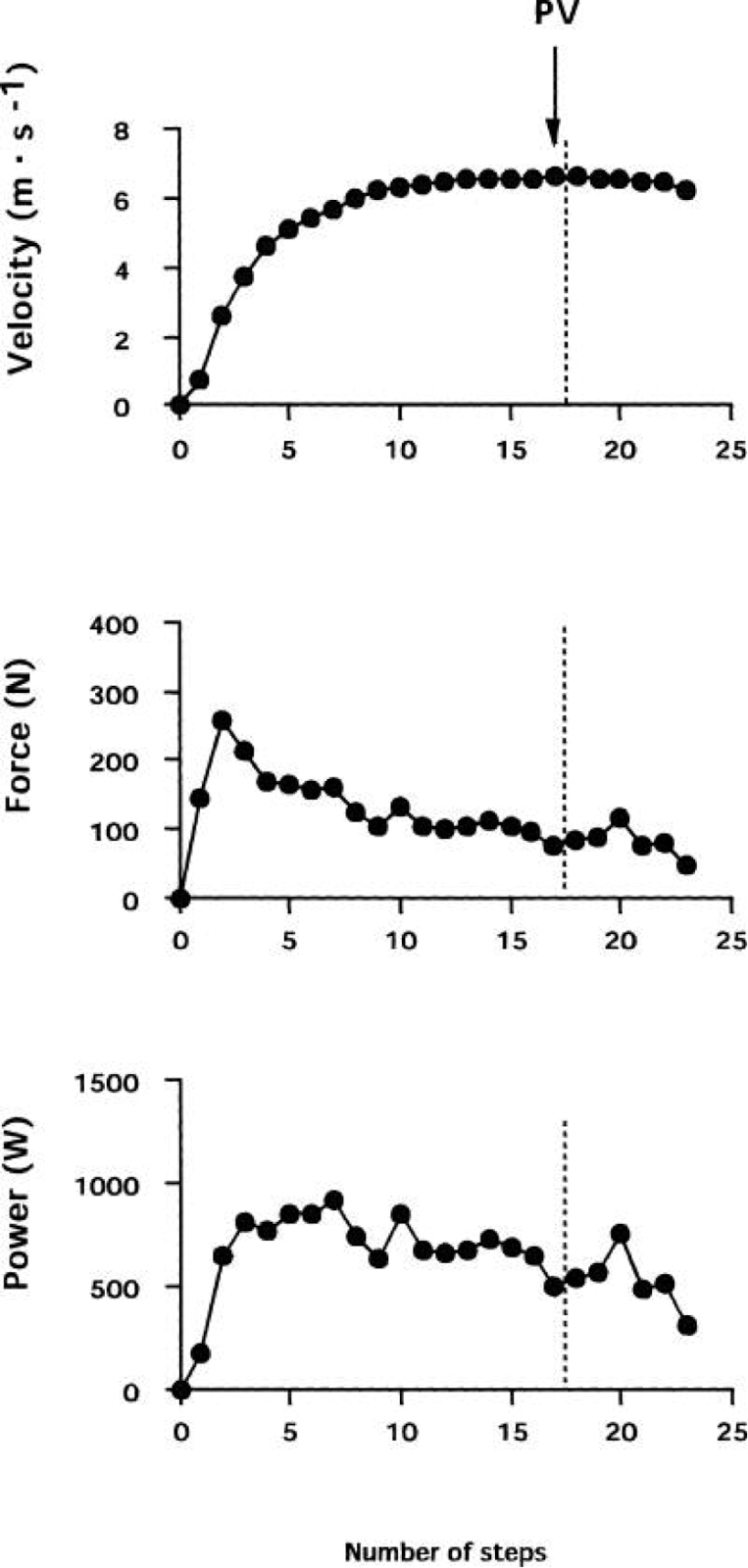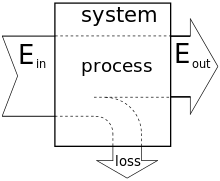## Sunday, 18 January 2015

### Numbers, numbers, everywhere \$#%# numbers

Passing from the Christmas sales straight to New Year's resolution territory come more gadgets for sports than ever. My sister-in-law got one of those 'fit bits' for xmas, I got a new watch, and loads of people out there are testing GPS devices some variety and figuring out what to do with them.

My god, what do we need some of these measurement things for? Let us first enumerate the variables that need (or more specifically can) be measured by a hand/foot held device, in order of utility:

1) Time
2) Distance
3) Speed
4) Heart Rate
5) Other stuff (not less useful)

Preceding my feelings on the matter, Alex Hutchinson has posted about new measuring devices on his Sweat Science blog titled Data Overload. The sentence that perhaps sums Alex's feelings best is: "There's no doubt you gain something with more data, but I think you also lose something".

Venturing into category five is a company titled Stryd, which boasts the ability to measuring power output of runners in real time:From Yanagiya et al. [here]From Stryd [here]

I am suspicious there are calibration issues with Stryd. While the above study shows that we might expect up to about 500-1000 watts for a ~63 kg teen boy running at 6 m/s, Stryd measures only 200 watts at 5 m/s. More to the point, power is nearly proportional to speed, especially if measuring mean power for a given distance D run in time T.

I to quote from a review on the matter (here).
It has been determined that the energy requirement (c) to cover any given distance is essentially independent of velocity, is consistent within individual athletes, and is equivalent to between 3.6-4.2 J/(kg·m).
In other words, if the following formula is true for energy expenditure per unit mass:

E/m = c·D
Then is it also true that power P = E/T so that

Pavg/m = c·D/T = c·v

Where v is one's forward-moving velocity. To estimate one's power expenditure, then, just plug in some numbers. Let's say m = 65 kg, v = 5 m/s, and c = 3.9 J/(kg·m). Then

Pavg = (65 kg)*(5 m/s)*(3.9 J/kg·m) = 1268 Watts

This value is clearly much larger than what Stryd estimates. Now the above 1268 watts is what the body exerts in total, whereas muscular efficiency (the mechanical work done) is between 18-26%.In any system, no matter how idealized (including the 'perfect' runner), one expects some loss due to waste heat and other entropic elements. From wiki article on Thermal Efficiency
This makes sense, as car engines about about 35% efficient. Hence of the above power exerted, only about 22% might be transferred to the ground, or about 220 - 330 watts, which appears to conform to the above graph. But a good portion of a running stride is spent in mid-air. The relation between total average power and peak power, when measuring in pulsed values, is proportional to the total step time divided by ground contact time (GCT):

The push-off time is roughly 1 metre divided by velocity, tGCT = (1 meter)/v, and the step time is roughly the inverse of the 180 steps per minute rule-of-thumb, or R = 3 steps per second, so that tstep = 1/R = 1/3. Therefore at moderate speeds (v > 3 m/s) the formula becomes

Pmax/m = (v/3)*Pavg = c·v2/3

Again, for the same 65 kg person above the maximum power output during each stride is about

Pmax = (5/3)*1268W = 2113 W

At a minimum 18% muscular efficiency we have a mechanical power of at least 380 W for a typical runner, which is clearly higher than what Stryd predicts. [NB that for faster speeds, a runner's turnover rate increases as well; in fact R is proportional to v2. In the end Pmax is directly proportional to velocity at most racing speeds such that

What was the point of all these calculations? That it is easy to create a wealth of data without saying much about the actual runner or their training. Those familiar with the math can have some fun with the numbers, but really how does this feed back into training. Though personally pleasing to tackle the conversion of biomechanic work to mechanical power, which coacher are interested? How many watts did my athlete produce? Who cares? The basic concept of translating  Pavg from speed is borderline trivial, and a simple derivative of information we already had.

Perhaps what could amount to interesting is when comparing to Tour de France cyclists (measured by an ergometer). Top cyclists a flat stage will expend up to 364 W for a 10 min stage (60 kg individual). For a 60 kg runner moving at, say, 6.5 m/s for 10 min (top runners can do this), then we have a power output of 1521 W, or 335 W at 22% muscular efficiency. Almost the same number!  Where there's willpower, there's a way.